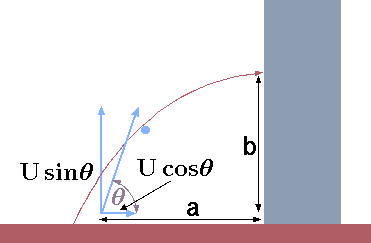# 3.2: Kinematics of a Point Body

$$\newcommand{\vecs}{\overset { \rightharpoonup} {\mathbf{#1}} }$$ $$\newcommand{\vecd}{\overset{-\!-\!\rightharpoonup}{\vphantom{a}\smash {#1}}}$$$$\newcommand{\id}{\mathrm{id}}$$ $$\newcommand{\Span}{\mathrm{span}}$$ $$\newcommand{\kernel}{\mathrm{null}\,}$$ $$\newcommand{\range}{\mathrm{range}\,}$$ $$\newcommand{\RealPart}{\mathrm{Re}}$$ $$\newcommand{\ImaginaryPart}{\mathrm{Im}}$$ $$\newcommand{\Argument}{\mathrm{Arg}}$$ $$\newcommand{\norm}{\| #1 \|}$$ $$\newcommand{\inner}{\langle #1, #2 \rangle}$$ $$\newcommand{\Span}{\mathrm{span}}$$ $$\newcommand{\id}{\mathrm{id}}$$ $$\newcommand{\Span}{\mathrm{span}}$$ $$\newcommand{\kernel}{\mathrm{null}\,}$$ $$\newcommand{\range}{\mathrm{range}\,}$$ $$\newcommand{\RealPart}{\mathrm{Re}}$$ $$\newcommand{\ImaginaryPart}{\mathrm{Im}}$$ $$\newcommand{\Argument}{\mathrm{Arg}}$$ $$\newcommand{\norm}{\| #1 \|}$$ $$\newcommand{\inner}{\langle #1, #2 \rangle}$$ $$\newcommand{\Span}{\mathrm{span}}$$$$\newcommand{\AA}{\unicode[.8,0]{x212B}}$$

A point body is location at time, $$t$$ in a location, $$\vec{R}$$. The velocity is derivative of the change of the location and using the chain role (for the direction and one for the magnitude) results, $\vec{U} = \frac{d\vec{R}}{dt} = \frac{d\vec{R}}{dt}|_{R} + \vec{\omega} \times \vec{R}$ Notice that $$\vec{\omega}$$ can have three dimensional components. It also can be noticed that this derivative is present derivation of any victory. The acceleration is the derivative of the velocity $\vec{a} = \frac{d\vec{U}}{dt} = \frac{d^{2}\vec{R}}{dt^{2}}|_{R} + \left(\vec{R} \times \frac{d\vec{\omega}}{dt}\right) + \vec{\omega} \times \left(\vec{R} \times \vec{\omega}\right) + 2\left(\frac{d\vec{R}}{dt}|_{R} \times \omega\right)$

Example 3.1

A water jet is supposed be used to extinguish the fire in a building as depicted in Figure 3.1. For given velocity, at what angle the jet has to be shot so that velocity will be horizontal at the window. Assume that gravity is $$g$$ and the distance of the nozzleFig. 3.1 Description of the extinguish nozzle aimed at the building window.

from the building is a and height of the window from the nozzle is $$b$$. To simplify the calculations, it proposed to calculate the velocity of the point particle to toward the window. Calculate what is the velocity so that the jet reach the window. What is the angle that jet has to be aimed?

Solution 3.1

The initial velocity is unknown and denoted as $$U$$ which two components. The velocity at $$x$$ is $$U_x = U \cos\theta$$ and the velocity in $$y$$ direction is $$U_y = U \sin\theta$$. There there are three unknowns, $$U$$, $$\theta$$, and time, $$t$$ and three equations. The equation for the $$x$$ coordinate is

$\label{mech:eq:sx} a = U\, \cos\theta \, t$

The distance for $$y$$ equation for coordinate (zero is at the window) is

$\label{mech:eq:sy} 0 = -\dfrac{g\,t^2}{2} + U\,\sin\theta\,t -b$ The velocity for the $$y$$ coordinate at the window is zero

$\label{mech:eq:uy} u (t) = 0 = -g\,t + U\,\sin\theta$ These nonlinear equations (3), (4) and (5) can be solved explicitly. Isolating $$t$$ from (3) and substituting into equations (3) and (5)

$\label{mech:eq:syn} b = \dfrac{-g\,a^2}{2\,U^2\,cos^2\theta} + a\,\tan\theta$ and equation (3) becomes

$\label{mech:eq:sxn} 0 = \dfrac{-g\,a}{U\,\cos\theta} + U\,\cos\theta \Longrightarrow U = \dfrac{\sqrt{a\,g}}{\cos \theta }$ Substituting (7) into (6) results in

$\label{mech:eq:theta} \tan\theta = \dfrac{b}{a} + \dfrac{1}{2}$